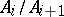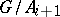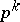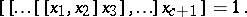# Nilpotent group

(diff) ← Older revision | Latest revision (diff) | Newer revision → (diff)

A group having a normal seriessuch that every quotientlies in the centre of(a so-called central series). The length of a shortest central series of a nilpotent group is called its class (or degree of nilpotency). In any nilpotent group the lower (and upper) central series (see Subgroup series) breaks off at the trivial subgroup (the group itself), and their lengths are equal to the nilpotency class of the group.

The finite nilpotent groups are exhausted by direct products of-groups, that is, groups of orders, whereis a prime number. In any nilpotent group the elements of finite order form a subgroup, the quotient group by which is torsion free. The finitely-generated torsion-free nilpotent groups are exhausted by the groups of integral triangular matrices with 1's along the main diagonal, and their subgroups. Every finitely-generated torsion-free nilpotent group can be approximated by a finite-group for every prime. Finitely-generated nilpotent groups are polycyclic groups (cf. Polycyclic group) and, moreover, have a central series with cyclic factors.

All nilpotent groups of class at mostform a variety (see Variety of groups), defined by the identityThe free groups of this variety are called free nilpotent groups. Concerning completions of torsion-free nilpotent groups see Locally nilpotent group.

How to Cite This Entry:
Nilpotent group. Encyclopedia of Mathematics. URL: http://encyclopediaofmath.org/index.php?title=Nilpotent_group&oldid=13294
This article was adapted from an original article by A.L. Shmel'kin (originator), which appeared in Encyclopedia of Mathematics - ISBN 1402006098. See original article